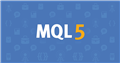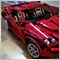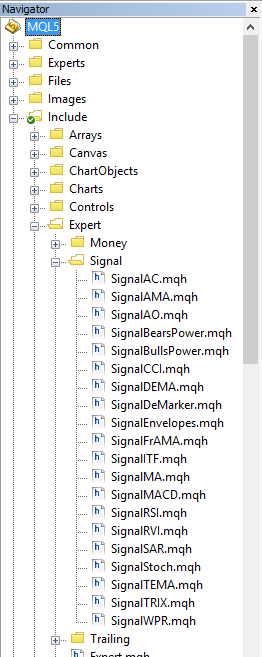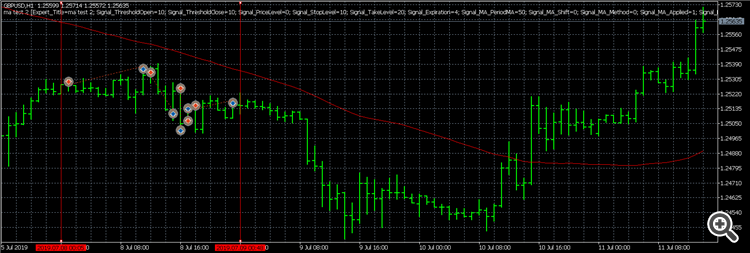# Intra time filter and threshold19

hello,

I am working on a EA  by using mql5 wizard generator. I did not really understand how the signal mechanism module and intra time filter works.

I need to open trades only in particular hours of the day. So as I understood bitmap for bad hours of the day I have to convert from binary to decimal. my excluding trading hours are 0h- 9h. so my bitmap in binary is 111111111100000000000000 and the decimal is 16760832.

When I gave this value and backtested my EA the trades the trades are still being opened in my excluding hours.

Next regarding mechanism of trade signals based on modules, I really don't understand what is the significance and how the threshold works.

https://www.mql5.com/en/docs/standardlibrary/expertclasses/csignal#mechanism

from the above link there is an example of two variants. Where did the significance value come from ?

Variant 1

The price crossed the rising MA upwards. This case corresponds to one of the market models implemented in the MA module. This model implies a rise of price. Its significance is equal to 100.At the same time, the Stochastic oscillator turned down and formed a divergence with price. This case corresponds to one of the models implemented in the Stochastic module. This model implies a fall of price. The weight of this model is 80.

Let's calculate the result of final "voting". The rate obtained from the MA module is calculated as 0.4 * 100 = 40. The value from the Stochastic module is calculated as 0.8 * (-80) = -64. The final value is calculated as the Arithmetic mean of these two rates: (40 - 64)/2 = -12. The result of voting is the signal for selling with relative strength equal to 12. The threshold level that is equal to 20 is not reached. Thus a trade operation is not performed.

for second variant  in example ,the significance value is 10. where are these coming from?Documentation on MQL5: Standard Library / Strategy Modules / Modules of Trade Signals
• www.mql5.com
The standard delivery of the client terminal includes a set of ready-made modules of trade signals for "MQL5 Wizard". When creating an Expert Advisor in MQL5 Wizard, you can use any combination of the modules of trade signals (up to 64). The final decision on a trade operation is made on the basis of complex analysis of signals obtained from...19170

pbzf: I need to open trades only in particular hours of the day. So as I understood bitmap for bad hours of the day I have to convert from binary to decimal. my excluding trading hours are 0h- 9h. so my bitmap in binary is 111111111100000000000000 and the decimal is 16760832.

Likely the bitmap is 0h=1, 1h=2, 2h=4 … so you want (0-9 inclusive) binary 1111111111 = 1023.19

Thanks I understood the bit map for the intraday filter.

I am still not able to understand the  significance of the model. I don't understand where that value is coming from.

The price crossed the rising MA upwards. This case corresponds to one of the market models implemented in the MA module. This model implies a rise of price. Its significance is equal to 100. At the same time, the Stochastic oscillator turned down and formed a divergence with price. This case corresponds to one of the models implemented in the Stochastic module. This model implies a fall of price. The weight of this model is 80.

Let's calculate the result of final "voting". The rate obtained from the MA module is calculated as 0.4 * 100 = 40. The value from the Stochastic module is calculated as 0.8 * (-80) = -64. The final value is calculated as the Arithmetic mean of these two rates: (40 - 64)/2 = -12. The result of voting is the signal for selling with relative strength equal to 12. The threshold level that is equal to 20 is not reached. Thus a trade operation is not performed.

Variant 2

The price crossed the rising MA downwards. This case corresponds to one of the models implemented in the MA module.This model implies a rise of price. Its significance is equal to 10. At the same time, the Stochastic oscillator turned down and formed a divergence with price. This case corresponds to one of the models implemented in the Stochastic module. This model implies a fall of price. The weight of this model is 80.

I have highlighted what I dint understand from the mql5 mechanism. In both variants the MA are giving signals and the significance changes. Any idea how the highlighted value is coming ? please I am stuck without understand that.750

pbzf:

Thanks I understood the bit map for the intraday filter.

I am still not able to understand the  significance of the model. I don't understand where that value is coming from.

The price crossed the rising MA upwards. This case corresponds to one of the market models implemented in the MA module. This model implies a rise of price. Its significance is equal to 100. At the same time, the Stochastic oscillator turned down and formed a divergence with price. This case corresponds to one of the models implemented in the Stochastic module. This model implies a fall of price. The weight of this model is 80.

Let's calculate the result of final "voting". The rate obtained from the MA module is calculated as 0.4 * 100 = 40. The value from the Stochastic module is calculated as 0.8 * (-80) = -64. The final value is calculated as the Arithmetic mean of these two rates: (40 - 64)/2 = -12. The result of voting is the signal for selling with relative strength equal to 12. The threshold level that is equal to 20 is not reached. Thus a trade operation is not performed.

Variant 2

The price crossed the rising MA downwards. This case corresponds to one of the models implemented in the MA module.This model implies a rise of price. Its significance is equal to 10. At the same time, the Stochastic oscillator turned down and formed a divergence with price. This case corresponds to one of the models implemented in the Stochastic module. This model implies a fall of price. The weight of this model is 80.

I have highlighted what I dint understand from the mql5 mechanism. In both variants the MA are giving signals and the significance changes. Any idea how the highlighted value is coming ? please I am stuck without understand that.

The values are hard-coded. Here's the one for stochastic (highlighted in green):

```CSignalStoch::CSignalStoch(void) : m_periodK(8),
m_periodD(3),
m_period_slow(3),
m_applied(STO_LOWHIGH),
m_pattern_0(30),
m_pattern_1(60),
m_pattern_2(50),
m_pattern_3(100),
m_pattern_4(90)
{
//--- initialization of protected data
m_used_series=USE_SERIES_OPEN+USE_SERIES_HIGH+USE_SERIES_LOW+USE_SERIES_CLOSE;
}
```

If you look into the corresponding CSignal files of individual models, you'll see the values that were hard-coded in their constructors.19

Seng Joo Thio:

The values are hard-coded. Here's the one for stochastic (highlighted in green):

If you look into the corresponding CSignal files of individual models, you'll see the values that were hard-coded in their constructors.

thanks for replying. can you please tell me in detail where I can find that csignal files? I am not able to find it.750

pbzf:

thanks for replying. can you please tell me in detail where I can find that csignal files? I am not able to find it.

Here:19

Seng Joo Thio:

Here:

Thanks a lot. But still I am not getting clear about it. from the Csignal of MA I get this data

CSignalMA::CSignalMA(void) : m_ma_period(12),
m_ma_shift(0),
m_ma_method(MODE_SMA),
m_ma_applied(PRICE_CLOSE),
m_pattern_0(80),
m_pattern_1(10),
m_pattern_2(60),
m_pattern_3(60)

what is the pattern 0 ? there are only 3 patterns for MA signals. I checked for other modules like stoch and RSI and there is a pattern 0 with significance.

And consider this example as I am not understanding threshold open and threshold close properly.  My weight of MA is 1.0 and the pattern 1 with significance of 10 occurs , then my threshold value is 1* 10=10 right?

now if I give threshold  open and close as 5 and 8 no trades should be opened. Isnt it correct? I generated this as  expert advisor but trades are being opened.  Please do clarify. It will be of great help.19

I also have a new issue. Its more of continuity of the threshold topic. I created EA with Moving average trading signal module from mql5 wizard generator.

The  only signal in the EA module is simple moving average with period 10. open and close threshold is 10 and 10. weight of the MA is 1.0.

I backtested it but I am getting results otherwise. check it out from the image below.((

the chart is from backtesting. the duration inside vertical redlines is the duration. According to the trading signal module of moving averages, price did not even touch or cross the moving average. (As seen from chart). so no trades should be opened in the period I have tested. But still trades are opened and its also in chart as the plotted arrows. I really don't understand this.  Can anyone explain this???750

pbzf:

Thanks a lot. But still I am not getting clear about it. from the Csignal of MA I get this data

CSignalMA::CSignalMA(void) : m_ma_period(12),
m_ma_shift(0),
m_ma_method(MODE_SMA),
m_ma_applied(PRICE_CLOSE),
m_pattern_0(80),
m_pattern_1(10),
m_pattern_2(60),
m_pattern_3(60)

what is the pattern 0 ? there are only 3 patterns for MA signals. I checked for other modules like stoch and RSI and there is a pattern 0 with significance.

And consider this example as I am not understanding threshold open and threshold close properly.  My weight of MA is 1.0 and the pattern 1 with significance of 10 occurs , then my threshold value is 1* 10=10 right?

now if I give threshold  open and close as 5 and 8 no trades should be opened. Isnt it correct? I generated this as  expert advisor but trades are being opened.  Please do clarify. It will be of great help.

You can look into the individual signals file to see what pattern 0 is for. It might have been the cause of your next post :).

As for thresholds, if you want less opens and closes, you should set their threshold to higher values - check ExpertSignal.mqh.750

pbzf:

I also have a new issue. Its more of continuity of the threshold topic. I created EA with Moving average trading signal module from mql5 wizard generator.

The  only signal in the EA module is simple moving average with period 10. open and close threshold is 10 and 10. weight of the MA is 1.0.

I backtested it but I am getting results otherwise. check it out from the image below.

((

the chart is from backtesting. the duration inside vertical redlines is the duration. According to the trading signal module of moving averages, price did not even touch or cross the moving average. (As seen from chart). so no trades should be opened in the period I have tested. But still trades are opened and its also in chart as the plotted arrows. I really don't understand this.  Can anyone explain this???

See my earlier post - could be due to a combination of pattern 0 and low threshold... you can turn it off and increase your thresholds for testing by adding this line in your expert:

```   signal.PatternsUsage(-2);
```